Published: 06 October 2023

# Research on road damage recognition and classification based on improved VGG-19

Jiaqi Wang1
Kaihang Wang2
Kexin Li3
1, 2Landscape Architecture, Beihua University, Jilin, China
3Beihua University, Jilin, China
Corresponding Author:
Jiaqi Wang
Article in Press
Views 28

#### Abstract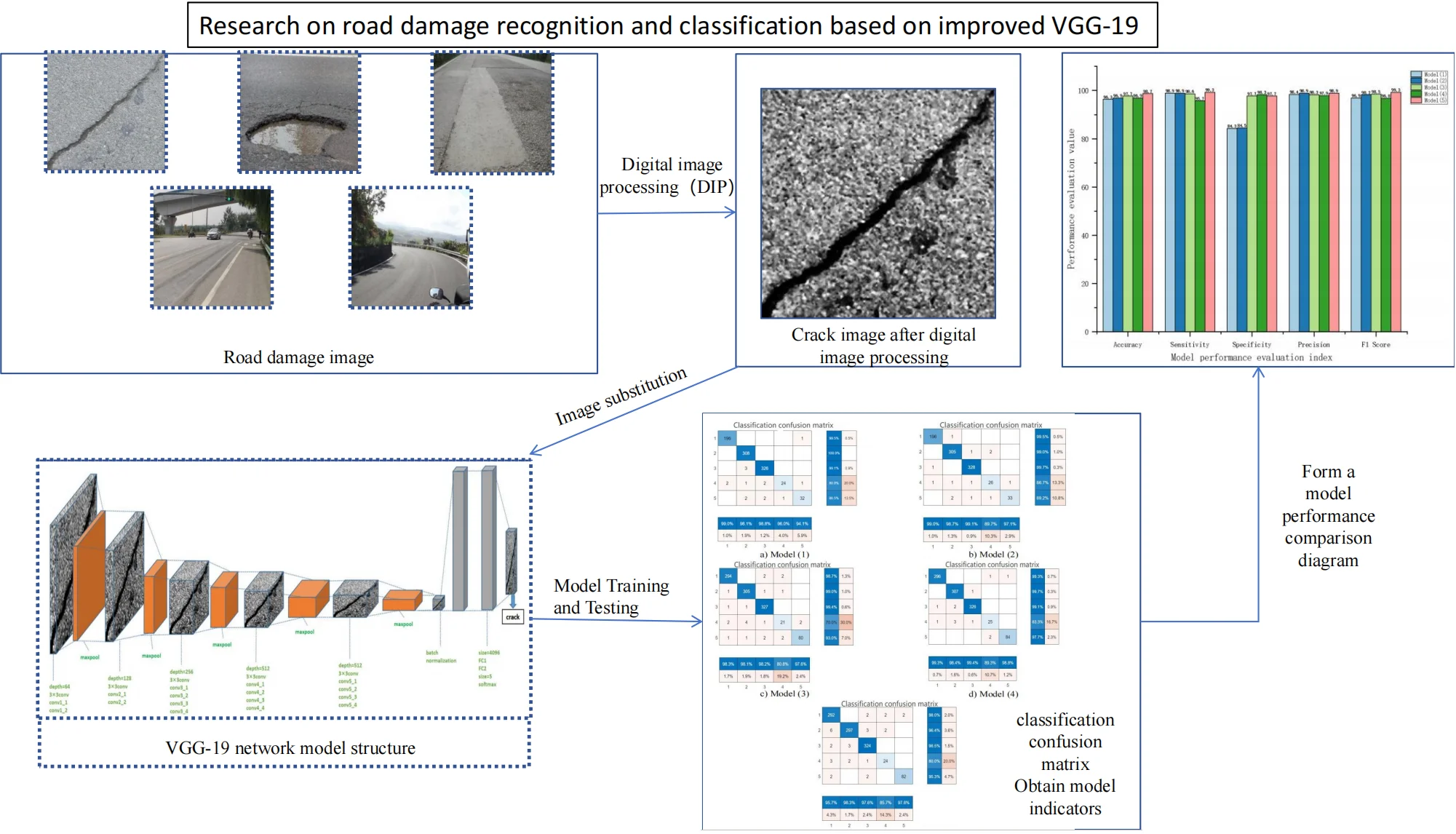#### Highlights

• Starting from the recognition performance of VGG-19 neural network model for pavement diseases, a total of five structural improvements were made.
• Using digital image processing technology, the pavement disease image is processed by noise reduction and brightness improvement.
• The classification confusion matrix and neural network model evaluation index are used to evaluate the model, and the Road damage VGG-19 model in this paper is obtained by comparative analysis.

## 1. Introduction

Whether it is concrete road or asphalt road, after a period of driving, various damages, deformations and other defects will occur one after another . The generation and extension of road damages will reduce the performance and life of road . At the same time, road damage can also cause serious traffic jams and accidents, resulting in adverse social impacts. Efficient and accurate recognition, detection and classification of road damages are the focus of researchers .

Therefore, this paper studies the classification method of road damage recognition based on improved VGG-19. The road damage image samples are obtained by network extraction and camera equipment shooting. Digital Image Processing (DIP) such as Region of Interest (ROI) and Histogram Equalization (HE) were used to remove the noise interference under the influence of environmental factors and obtain the gray image of road damages. In the case of limited sample labels, a VGG-19 network model for identification and classification of various road damages is established based on MATLAB R2022b. Based on statistical data analysis methods, the performance indicators of each network model are sorted and analyzed. On this basis, the VGG-19 network model which can accurately and efficiently train, identify and classify road damages is obtained through experimental comparison.

## 2. Road damage image processing based on improved VGG-19

In order to realize the accurate identification of road damage in the case of a small number of samples, the VGG-19 network model is constructed based on MATLAB. It is necessary to process the obtained road damage images to make the illumination on the images uniform, reduce noise interference, eliminate irrelevant information in the images, restore useful real information, enhance the detectability of relevant information and simplify the data to the greatest extent, obtain samples that can effectively identify road damage parameter information, and construct a VGG-19 network model .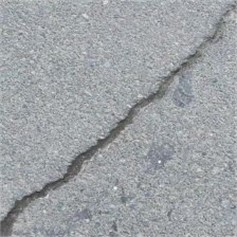a) Crack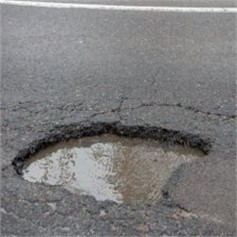b) Pothole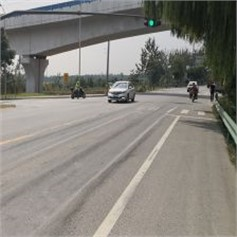c) Rut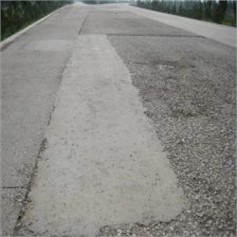d) Loose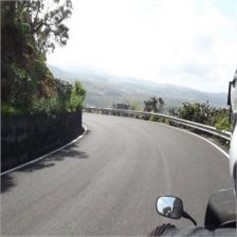e) Normal

The road surface damage contained in Fig. 1 are analyzed. The contrast is blurred, and the unique texture features of various damage types are not obvious. They are seriously disturbed by environmental noise such as automobiles and illumination. Therefore, in order to better fit the VGG-19 network model, it is necessary to perform image denoising and image enhancement processing on road damage images.

### 2.2. Road damage image processing

The quality of the directly obtained pavement disease image is poor, and the network model is difficult to capture the feature information of the disease image . In order to make the pavement disease image better fit the VGG-19 network model, eliminate the redundant noise interference, and enhance the feature information beneficial to the network model training in the pavement disease image, the pavement disease image is processed based on image processing methods such as gray level changes (GLC) normalization (Normalize), region of interest extraction (ROI), normalization (Normalize), histogram equalization (HE), and median filtering (MF) to obtain pavement disease images with strong recognition, high contrast, and obvious texture features. Improve the efficiency of VGG-19 classification and recognition images. The image processing flow of pavement disease in this study is shown in Fig. 2.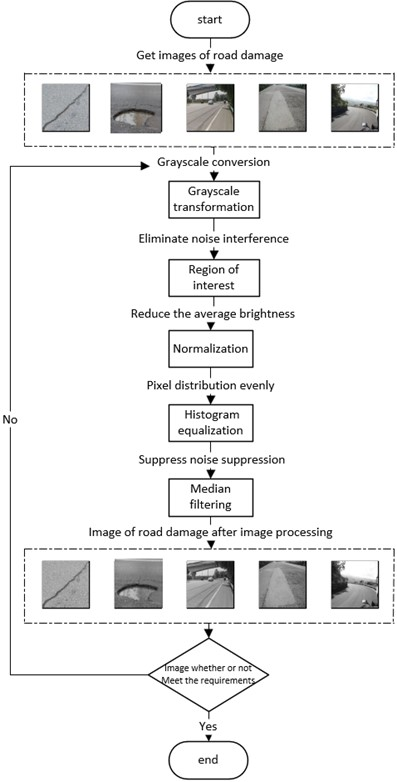### 2.2.1. Gray level change

The gray level transformation of the image refers to the method of changing the gray value of each pixel in the source image point by point according to a certain transformation relationship according to a certain target condition. The purpose is to improve the image quality and make the image display effect clearer. The gray-scale transformation method used in the research is a gray-scale linear transformation, which belongs to a kind of gray-scale transformation. By establishing a gray-scale mapping to adjust the gray level of the source image, the purpose of image enhancement is achieved. The principle is to enhance or weaken the gray level of the image by transforming the pixel value of the image through a specified linear function. The formula of linear transformation of gray scale is a common one-dimensional linear function, as shown:

1
$g\left(x,y\right)=k\cdot f\left(x,y\right)+b.$

Let $x$ be the original gray value, then the calculation formula of the gray value of the transformed $y$ is as shown:

2
$y=k\cdot x+b,\left(0\le y\le 255\right),$

where $k$ represents the slope of the line, that is, the degree of tilt, $b$ represents the intercept of the linear function in the $y$-axis. In the formula, the values of different $k$ and $b$ represent different meanings: when $k>$ 1, it means the contrast of the enhanced image, the pixel value of the image increases after linear transformation, and the overall effect is enhanced. When $k=$ 1, it means that the image brightness can be adjusted by adjusting the size of $b$. When 0 $ 1, the overall contrast of the image is weakened; when $k<$ 0, it means that the bright area in the original image darkens and the dark area in the original image brightens.

### 2.2.2. Region of interest extraction

After converting road damage image into a grayscale image, the image part containing the texture features of the road damage is set as the “region of interest” (ROI) of the image. ROI interception is a very critical step in image processing. The interception of ROI region can reduce the amount of data in subsequent image processing while eliminating some noise. The study selected a window of 2440×2600 to intercept the ROI region of five road damage images.

### 2.2.3. Road damage image normalization

In the process of image processing, image normalization is a necessary preprocessing process. There are three purposes to study image normalization. First, if image normalization is not performed, the size of the image cannot perfectly fit the input layer size of the VGG-19 network model, resulting in the network model cannot be trained. Second, if the input layer size is set to be large, the gradient transmitted to the input layer will become large during back propagation. If the gradient is large, a smaller learning rate must be set, otherwise it will cross the optimal. In this case, the selection of the learning rate needs to refer to the size of the input layer value, and the data normalization operation can easily select the learning rate, so as to facilitate the network model training ; third, image normalization can remove the average brightness value of the image. Subtracting the statistical average of the data on each image can remove the common part and highlight individual differences.

Based on a common image normalization principle, the road damage image is normalized (Normalize) batch processing. The principle is shown in Eq. (3):

3
$y=\frac{x-MinValue}{MaxValue-MinValue}.$

Among them, $x$ and $y$ are the values before and after normalization, respectively. MaxValue and MinValue are the maximum and minimum gray values of the original image, respectively. On this basis, the image size is modified to 244×244 to perfectly match the input layer of the network model.

### 2.2.4. Histogram equalization

Histogram plays an important role in image processing. The gray histogram of the image describes the gray distribution in the image, which can intuitively show the amount of each gray level in the image. Histogram equalization (HE) is a method to enhance the contrast of images. The main idea is to transform the histogram distribution of an image into an approximate uniform distribution through the cumulative distribution function, thereby enhancing the contrast of the image. In order to extend the brightness range of the original image, a mapping function is needed to evenly map the pixel values of the original image to the new histogram, but the mapping function has two necessary conditions : First, the order of the original pixel values cannot be disrupted, and the size relationship between bright and dark after mapping cannot be changed; second, the mapping must be in the original range, that is, the range of the pixel mapping function should be between 0 and 255.

Since the image is composed of pixels, the image histogram equalization is solved by the discrete cumulative distribution function. In the process of histogram equalization, the mapping method is shown in Eq. (4):

4
$Sk={\sum }_{j=0}^{k}\frac{nj}{n},\mathrm{}\mathrm{}\mathrm{}\mathrm{}\mathrm{}\left(k=0,1,2,...,L-1\right).$

Among them, ${S}_{k}$ refers to the value of the current gray level after the cumulative distribution function mapping, $n$ is the sum of pixels in the image, ${n}_{j}$ is the number of pixels in the current gray level, and $L$ is the total number of gray levels in the image.

### 2.2.5. Median filtering

The median filtering (MF) is a nonlinear smoothing image processing technology, which is a nonlinear image processing technology based on the order statistics theory and can effectively suppress noise. The basic principle of median filtering is to replace the value of a point in a digital image or digital sequence with the median value of each point in a neighborhood of the point, so that the surrounding pixel values are close to the true value, thereby eliminating isolated noise points.

Based on the two-dimensional median filtering function (medfilt2) of MATLAB, the median filtering (MF) processing of road damage images is carried out by filling the image edges, adding salt and pepper noise, and data conversion. Set the one-dimensional sequence ${f}_{n}$, take the window length $m$ ($m$ is odd), and perform median filtering on it. The number of $m$ is successively taken out from the input sequence, and the number of m is sorted by size, and the number whose serial number is the center point is taken as the filter output. The principle is as shown in Eq. (5):

5
$\begin{array}{c}y\left(i\right)=Med\left\{f\left(i-v\right),......,f\left(i\right),......,f\left(i+v\right)\right\},i\in N,v=\frac{m-1}{2}.\end{array}$

Taking the road crack image as an example, the road crack image after image processing is shown in Fig. 3.

Fig. 3Final image of road cracks after image processing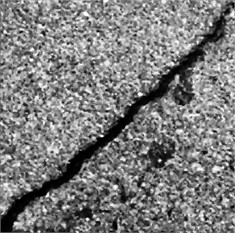By analyzing the road crack image information in Fig. 3, it can be seen that the crack image processed by image processing methods such as gray level changes (GLC), region of interest extraction (ROI), normalization (Normalize), histogram equalization (HE) and median filtering (MF) has uniform illumination, the contrast of interference noise is significantly reduced, and the contrast of cracks is significantly increased. This image can perfectly fit the training and recognition of the neural network model. This road damage image can form a road damage image data set for use by the neural network model.

## 3. Establishment of road damage recognition model based on VGG-19

### 3.1. Improved methods of VGG-19 model

In convolutional neural networks, VGG-19 is often used in the field of object recognition. Each layer of the VGG-19 neural network will use the output of the previous layer to further extract more complex features until it is complex enough to be used to identify objects, so each layer can be seen as many local feature extractors. However, there are still some shortcomings and defects in the identification of road damages. The ordinary VGG-19 network model often appears in the training and testing of neural networks, such as slow speed, low training accuracy and over-fitting. In order to solve the above problems, combined with the characteristics of road damage images, an improved model based on VGG-19 network model structure is proposed: Road damage VGG-19.

The construction of Road damage VGG-19 network model adopts the method of adjusting batch size and adding batch normalization layer. Because the most important factor affecting the training speed and test accuracy of the VGG-19 network model is the size of the batch size, the batch size can be defined as the number of samples taken in one training, which belongs to a hyperparameter, usually set when the model is trained, generally 2$n$. The size of the Batch size affects the optimization degree and speed of the model. At the same time, it directly affects the use of GPU memory. Too large or too small Batch size has a great impact on the model. Therefore, it is necessary to set an appropriate batch size value to find the best balance between training speed and memory capacity. In the training process of the neural network, as the depth deepens, the input value distribution will shift to the upper and lower ends of the value interval, which leads to the disappearance of the gradient of the low-level neural network during back propagation, which is an important reason for the slower convergence of the deep network. The batch Normalization can force the distribution of the input values of each layer of the neural network back to the standard normal distribution with a mean value of 0 and a variance of 1 through a certain standardization method. Its role can accelerate the convergence speed of the model training, so that the model can detect and identify the road damage image faster and more accurately with a small number of sample labels. The Road damage VGG-19 network model is obtained by adding the batch normalization layer, and its network structure is shown in Fig. 4.

By analyzing the network structure shown in Fig. 4, it can be seen that the road damage image enters the VGG-19 network input layer as input data. After five convolution layers, five pooling layers, data normalization, and full connection layers, the network model recognizes the image as a crack image. Then, the final output layer of the VGG-19 network model outputs the image as a road crack image.

Fig. 4Road damage VGG-19 network structure diagram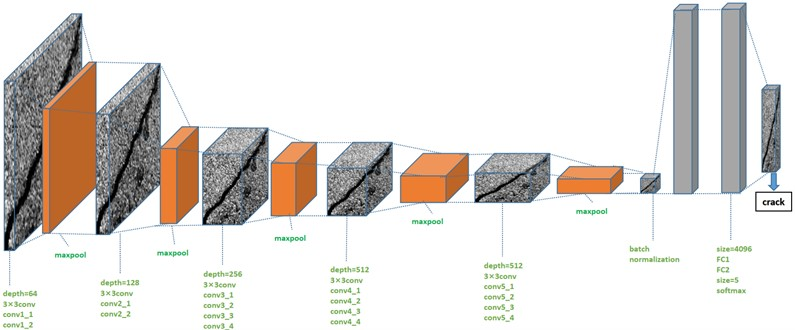Table 1Improved VGG-19 network model training information

 Model serial number Training time Accuracy of validation Model (1) 210 min 28 s 94.79 % Model (2) 185 min 25 s 94.79 % Model (3) 202 min 05 s 92.89 % Model (4) 186 min 20 s 94.31 % Model (5) 44 min 41 s 97.20 %

By analyzing the data in Table 1, it can be seen that Model (5) has much higher efficiency than other models in pavement disease identification, and the verification accuracy of Model (5) for road damage images is higher than that of other models. It can be seen that the ability of Model (5) to recognize road damage images is higher than other models. Therefore, Model (5) is used as the VGG-19 network model of this study, and it is named Road damage VGG-19 road damage identification model. In addition, according to the type of road damage recognition during model training, the final output size of the model is defined as 5.

### 3.2. Improved VGG-19 network model training

The configuration of the running device is one of the main factors affecting the training time of the model, that is, the CPU and GPU of the device. Experimental environment: CPU: AMD Ryzen 7 6800H with Radeon Graphics, 3.20 GHz; gPU: NVIDIA GeForce RTX3060 Lap Top GPU, combined with MATLAB deep learning framework, completed training in MATLAB R2022b environment.

In order to improve the training speed and verification accuracy of the model, the matching ability and recognition ability of the model training for the road damage image are strengthened. The training parameters of the model (1-5) are uniformly set to 64 mini batch size, 0.0001 Initial Learn Rate and 30 Validation Frequency. The setting of the mini batch size can divide the road damage image sample set into equal subsets, and train each subset to improve the training speed of the road damage recognition model. Setting the initial learning rate reasonably can make the road damage recognition model converge to the local minimum in a suitable time when training and recognizing the road damage image, so as to improve the training speed of the road damage recognition model. In addition, reasonably setting the number of iterations (Validation Frequency) can prevent the over-matching problem of the road damage recognition model when training and recognizing the road damage image.

In addition to the above unified training parameters, the fundamental difference between model (1-5) training is whether the batch normalization layer and the position of the batch normalization layer are added, which determines the speed of training time and the accuracy of training. The specific settings can be seen in Table 1 in Section 2.1.

After the VGG-19 network model training is completed, the trained model parameters are saved for subsequent model testing.

### 3.3. Improved VGG-19 network model testing

In this study, in the MATLAB R2022b test environment, after training the model (1-5), the trained model parameters are loaded, and the accuracy of classification and recognition is obtained by model test classification. In the test set, the cracks, potholes, ruts, loose and normal roads are tested and identified in turn, and the average time and accuracy of each road damage identification test are counted to form the model (1-5) test data comparison table, see Table 2.

Table 2Improved VGG-19 network model test information

 Model serial number Average test time Accuracy Model (1) 0.59 s 97 % Model (2) 0.45 s 98 % Model (3) 0.69 s 98 % Model (4) 0.47 s 99 % Model (5) 0.19 s 98 %

By analyzing the data in Table 2, it can be seen that average test time of model (1-4) is more than 0.45 s. However, the average test time of model (5), namely Road damage VGG-19 road damage identification model, is only 0.19 s, and the average test time of Road damage VGG-19 road damage identification model is the shortest. Secondly, the test accuracy of Road damage VGG-19 road damage recognition model is 98 %, which is the second in all test models, which is in line with the significance of model modification, and has played a role in improving the accuracy of the model and reducing the test time.

It can be concluded that the efficiency, verify accuracy and average test time of Model (5), that is, Road damage VGG-19 pavement disease recognition model, are better than other models.

## 4. Feasibility evaluation of road damage VGG-19 network model

### 4.1. Construction of network model performance evaluation index

In order to verify the effectiveness of the network model, the feasibility of model (1-5) is evaluated after the training and testing stages are completed. Performance evaluation indicators such as Accuracy, Sensitivity, Specificity, Precision, and F1 Score were used to verify the performance of the model. These values depend on the true positive (TP), false negative (FN), true negative (TN) and false positive (FP) values, which can be calculated from the confusion matrix of the five models. The calculation formula and corresponding description of the performance index are as follows:

6
$Accuracy=\frac{TP+TN}{TP+FN+TN+FP},$
7
$Sensitivity=\frac{TP}{TP+FN},$
8
$Specificity=\frac{TN}{TN+FP},$
9
$Precision=\frac{TP}{TP+FP},$
10
$F1Score=\frac{2TP}{2TP+FN+FP}.$

Accuracy represents the proportion of all samples with correct prediction in all test samples, reflecting the recognition accuracy of road damage recognition model for road damage images. Sensitivity, also known as recall rate, represents the proportion of correct prediction results in all positive events, reflecting the recognition ability of road damage recognition model for road damage images. Specificity expresses the recognition ability of negative samples, which is similar to sensitivity, and also reflects the recognition ability of road damage recognition model for road damage images. Precision represents the proportion of true positive samples in all predicted positive samples, reflecting the recognition accuracy of road damage recognition model for road damage images. F1 score. In the competition of some classification problems, F1 score is used as the final evaluation method, which represents the harmonic average of precision and recall. The maximum is 1 and the minimum is 0. For the road damage recognition model, F1 score is often used to reflect the performance of the model.

The essence of the classification confusion matrix is a table. Through the collection of samples, we can directly $k$ now the positive and negative situations of the predicted road damage images in the real situation . At the same time, the results of the classification model can be obtained from the sample data, and the Positive and Negative in the data can also be grasped. Therefore, four basic indicators are obtained, which are collectively referred to as the first-level indicators. The four indicators are presented in the table to obtain the following matrix, namely the classification confusion matrix, which defines TP, FN, TN and FP based on True, False, Positive and Negative. For the road damage recognition model, the classification confusion matrix reflects the number of observations of the road damage model for the road damage image misclassification and classification. The classification confusion matrix of (1-5) is shown in Fig. 5.

By analyzing the confusion matrix in Fig. 5, it can be seen that the output type of the network model is five types. Therefore, the classification confusion matrix of models (1-5) belongs to the multi-classification confusion matrix. In each classification confusion matrix, the real class and the prediction class are included. The real class represents the real value, and the prediction class represents the predicted value. The serial numbers 1-5 represent the cracks, potholes, rutting, loose and normal road images respectively. Taking the classification confusion matrix of Model (5) as an example, the blue number in the 5×5 matrix represents the correct number of road damages identified for each type. Taking the first column of the matrix as an example, it represents that 292 images are identified as road crack damages, 6 images are identified as road pothole damages, 2 images are identified as road rutting damages, 3 images are identified as road loose damages and 5 images are identified as normal road. The blue percentage below the first column represents the proportion of the damage with the real label as the road crack being correctly predicted, and the yellow percentage represents the proportion of the image with the real label as the road crack being incorrectly predicted as other types of road damages. According to the data obtained in the matrix, the values of TP, FN, TN and FP can be calculated, and then the evaluation indexes can be calculated.

Fig. 5Classification confusion matrix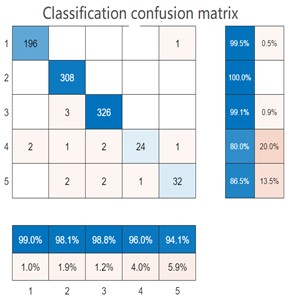a) Model (1)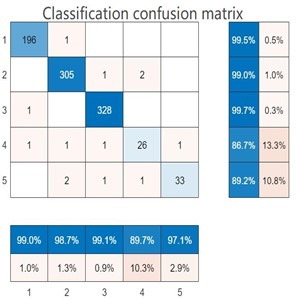b) Model (2)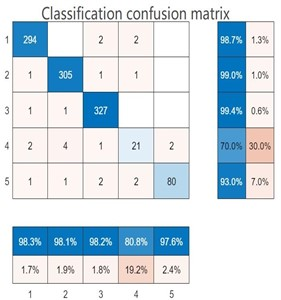c) Model (3)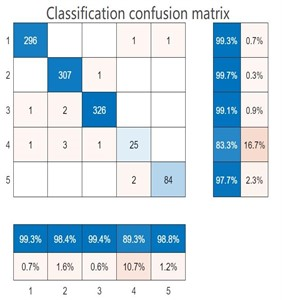d) Model (4)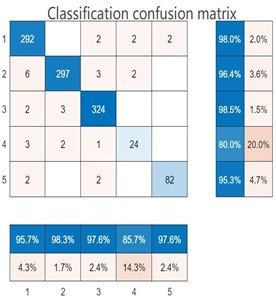e) Model (5)

### 4.2. Performance comparison of road damage recognition network models

According to the classification confusion matrix in Fig. 6, the values of TP, FN, TN and FP are calculated. TP, FN, TN and FP are all related to the recognition ability of the model to road damage images. The evaluation indexes were calculated by combining the Eqs. (6-10) in Section 3.1 with the values of TP, FN, TN and FP. The value of accuracy represents the recognition accuracy of the model for all types. However, other performance evaluation indicators represent the performance values of the model for a certain type of recognition. Therefore, this study corresponds other performance evaluation indicators to road crack identification .

In the performance evaluation of the model, the values of TP, FN, TN and FP are the first-level indicators, and the values of Accuracy, Sensitivity, Specificity and Precision calculated by them are the second-level indicators. On the basis of these four second-level indicators, a third-level indicator, F1 score, will be generated. The comparison of each evaluation index is shown in Fig. 6.

Fig. 6Comprehensive comparison chart of neural network model performance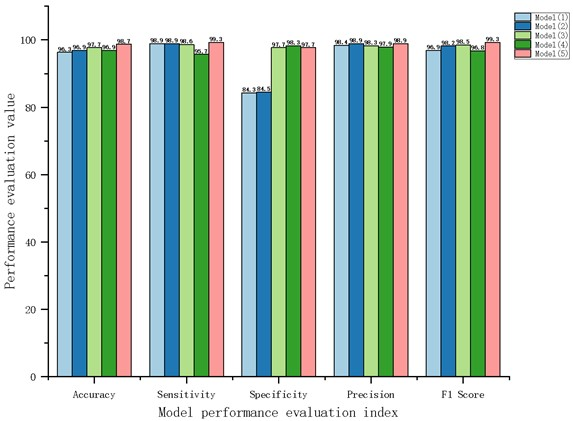By analyzing the image information in Fig. 6, it can be seen that the accuracy, sensitivity, precision and F1 score of Model (5), namely Road damage VGG-19 road damage recognition model, are higher than those of the other four models. However, the specificity is lower than the model (4). Through comprehensive analysis, the research improves the performance of the model by modifying the parameters and structure of the VGG-19 network model.

## 5. Conclusions

In the research, the problems existing in the current road damage detection and recognition are discussed, which may be caused by the model itself and the image itself. Therefore, the research first uses digital image processing techniques such as grayscale conversion, ROI interception, histogram equalization, and median filtering to remove environmental noise interference in road damage images, and obtain road damage images with high contrast and clear targets. Then, the research starts with optimizing the parameters and structural mechanism of the network model, and proposes a total of five road damage identification VGG-19 network model improvement schemes. The five models are trained and tested in turn. Finally, the Road damage VGG-19 road damage identification model with the shortest average test time (0.19 s) and the highest test accuracy (98 %) is selected as the final model. Finally, based on the first-level evaluation index of neural network model, the second-level and third-level indexes of performance evaluation are calculated, and the comprehensive performance evaluation of five road damage identification models is carried out.

The verification analysis shows that the accuracy, sensitivity, precision and F1 score of the road damage VGG-19 road damage recognition model proposed in this paper are higher than other improved models in the comprehensive performance evaluation link, and the comprehensive performance is improved by 2.4 % compared with the traditional VGG-19 network model. It is proved that the model performance of road damage recognition model can be improved by modifying the parameters and structure of VGG-19 neural network.

12 June 2023
Accepted
22 August 2023
Published
06 October 2023
Keywords
digital image processing
VGG-19
neural network model evaluation index
Acknowledgements

2023 Beihua University Graduate Program “Research on road damage recognition and classification based on improved VGG-19”,  058. 2022 Beihua College Students Innovation and Entrepreneurship Training Program Project “Development and Application of Pavement Damage Identification System Based on CNN”, Project No. 202210201115.

Data Availability

The datasets generated during and/or analyzed during the current study are available from the corresponding author on reasonable request.

Author Contributions

Jiaqi Wang performed the conceptualization, data Curation, formal Analysis, project Administration, software and writing; Kaihang Wang performed the validation and visualization; Kexin Li performed supervision.

Conflict of interest

The authors declare that they have no conflict of interest.